## 6.5.2 Davenport-Schinzel Sequences

Davenport-Schinzel sequences provide a powerful characterization of the structure that arises from the lower or upper envelope of a collection of functions. The lower envelope of five functions is depicted in Figure 6.42. Such envelopes arise in many problems throughout computational geometry, including many motion planning problems. They are an important part of the design and analysis of many modern algorithms, and the resulting algorithm's time complexity usually involves terms that follow directly from the sequences. Therefore, it is worthwhile to understand some of the basics before interpreting some of the results of Section 6.5.3. Much more information on Davenport-Schinzel sequences and their applications appears in . The brief introduction presented here is based on .For positive integersand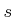, an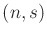Davenport-Schinzel sequence is a sequence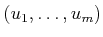composed from a set ofsymbols such that:

1. The same symbol may not appear consecutively in the sequence. In other words,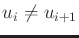for anysuch that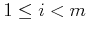.
2. The sequence does not contain any alternating subsequence that uses two symbols and has length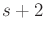. A subsequence can be formed by deleting any elements in the original sequence. The condition can be expressed as: There do not existindices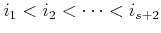for which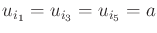and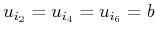, for some symbols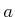and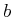.
As an example, an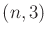sequence cannot appear as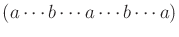, in which each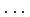is filled in with any sequence of symbols. Let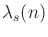denote the maximum possible length of anDavenport-Schinzel sequence.

The connection between Figure 6.42 and these sequences can now be explained. Consider the sequence of function indices that visit the lower envelope. In the example, this sequence is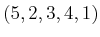. Suppose it is known that each pair of functions intersects in at mostplaces. If there arereal-valued continuous functions, then the sequence of function indices must be anDavenport-Schinzel sequence. It is amazing that such sequences cannot be very long. For a fixed, they are close to being linear.

The standard bounds for Davenport-Schinzel sequences are 6.9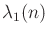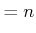(6.32)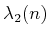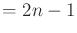(6.33)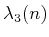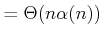(6.34)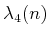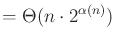(6.35)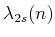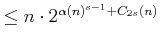(6.36)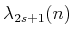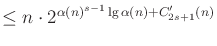(6.37)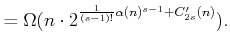(6.38)

In the expressions above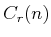andare terms that are smaller than their leading exponents. The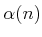term is the inverse Ackerman function, which is an extremely slow-growing function that appears frequently in algorithms. The Ackerman function is defined as follows. Let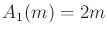and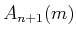represent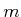applications of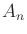. Thus,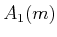performs doubling,performs exponentiation, and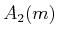performs tower exponentiation, which makes a stack of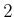's,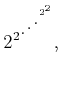(6.39)

that has height. The Ackerman function is defined as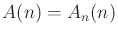. This function grows so fast that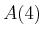is already an exponential tower of's that has height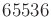. Thus, the inverse Ackerman function,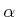, grows very slowly. Ifis less than or equal to an exponential tower of's, then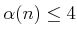. Even when it appears in exponents of the Davenport-Schinzel bounds, it does not represent a significant growth rate.

Example 6..9 (Lower Envelope of Line Segments)   One interesting application of Davenport-Schinzel applications is to the lower envelope of a set of line segments in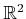. Since segments in general position may appear multiple times along the lower envelope, the total number of edges is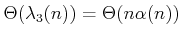, which is higher than one would obtain from infinite lines. There are actually arrangements of segments inthat reach this bound; see .Steven M LaValle 2020-08-14